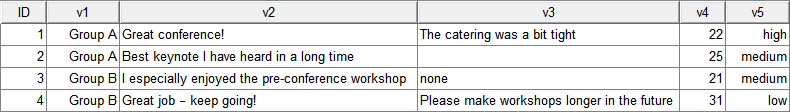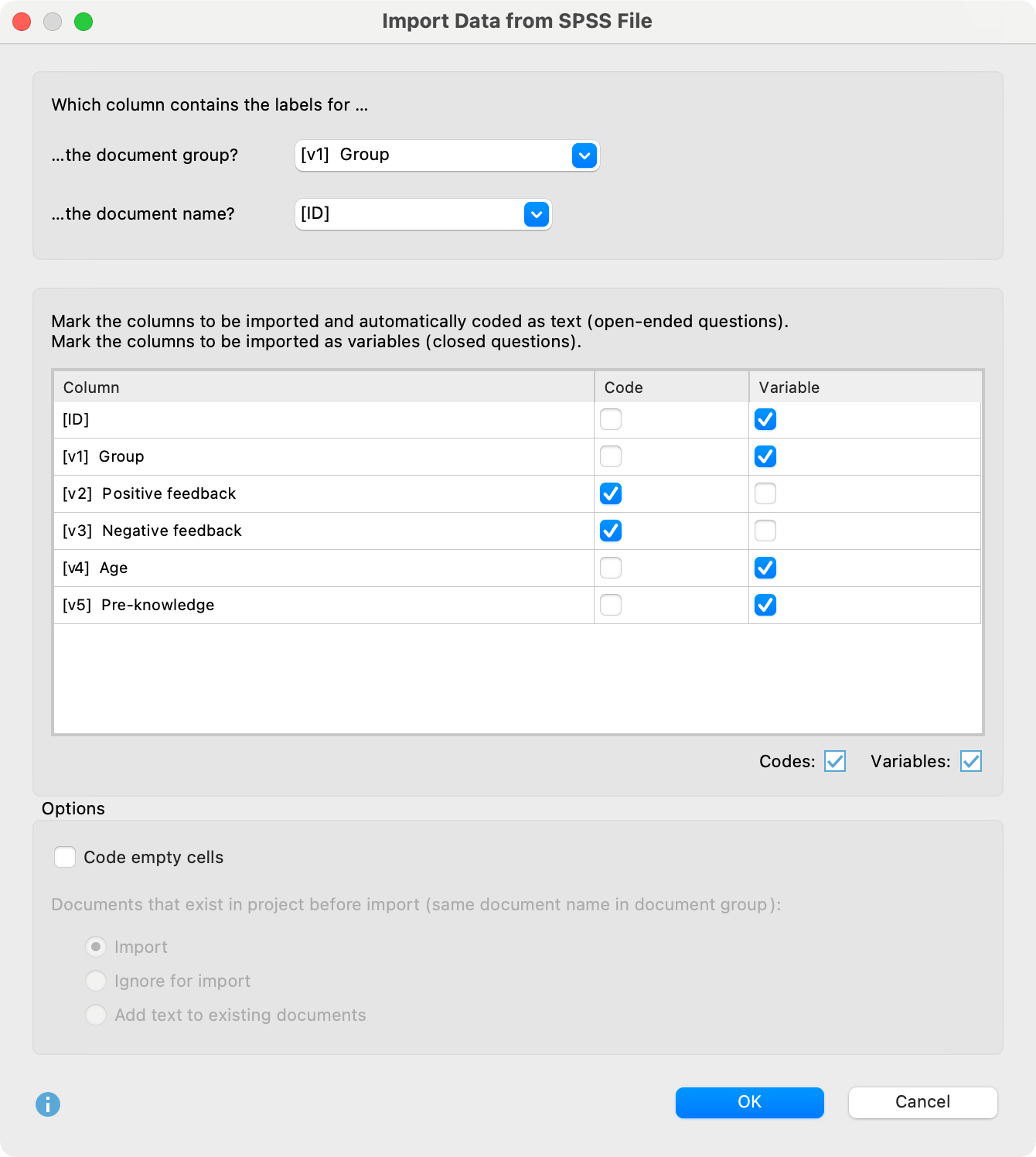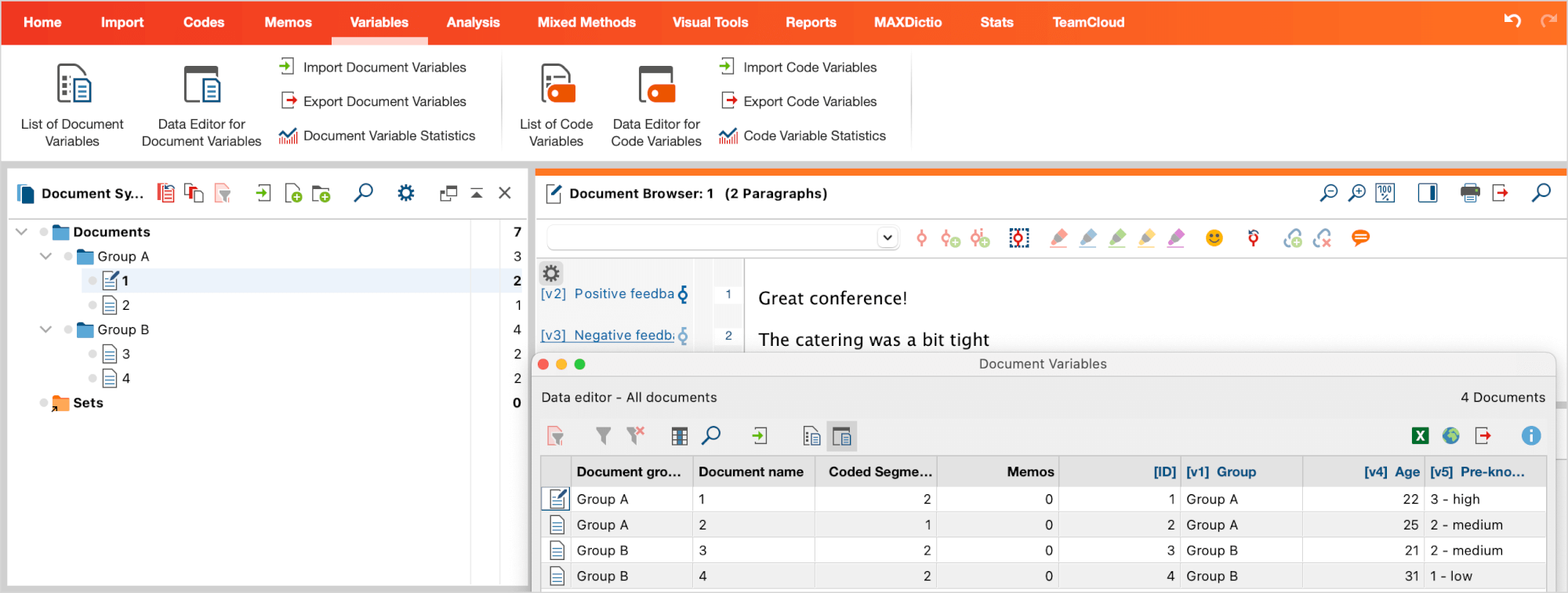# Survey Data from SPSS

You can import data sets from a survey that are available in an SPSS file into a MAXQDA project. During the import, a text document with the answers to open-ended questions is created for each case, while the standardized answers are saved as document variables. SPSS files have the following structure: the cases form the rows, and the columns contain the variables.## Start import

To import survey data from an SPSS file, call Import > Survey Data > Import Data from SPSS File from the menu:## Set options for import

After calling the function, the SPSS file must be selected in the file dialog. A window will then open in which you can specify settings for the import.The upper two settings determine which SPSS variables are to be used as document group and document name. MAXQDA automatically selects the first column of the data set as the document name.

Tip: When importing survey data to open-ended questions, it is advisable to choose the IDs of the respondents as document names, so that the clear assignment of answers to cases is guaranteed at all times – even when exporting data to statistical software at a later date.

MAXQDA does not automatically select an SPSS variable as “Document group”; instead, the entry Create new document group is initially selected in the drop-down menu. If you leave this default setting, all documents will automatically be imported into a new document group created by MAXQDA. If you select an SPSS variable instead, MAXQDA creates a document group for each value of this variable and assigns the data sets to the appropriate groups. In the image above, the variable v1 with variable label “Group” is selected.

In the middle area of the window, you can select which columns of the dataset should be imported as coded text and which as variables.

Note: When opening the dialog, MAXQDA has already marked all columns as coded text for import that have very different values, as these are most likely the answers to open questions.

If the check mark is set for both “Code” and “Variable”, MAXQDA imports the contents of this column as coded text and as a document variable. If neither “Code” nor “Variable” is checked, MAXQDA will ignore the data matrix column during import.

The options in the lower area are the same as for importing survey data from an Excel file and are described there in detail. The same applies to the options for the dialog that appears for importing the variables after clicking OK.

## Imported texts in MAXQDA

After importing the SPSS example data shown above into an empty project, the display in MAXQDA looks something like this (when opening the Data Editor for Document Variables):When importing, MAXQDA proceeds as follows:

• Each case gets its own text document.
• Document groups that do not yet exist are created.
• The variable names from the SPSS file including their labels are inserted as new top-level codes in the code system – if they do not exist. All text segments of the answers to open-ended questions are coded with the corresponding code. The complete variable label (which may be longer than the maximum length of code names) is include in the code memo for each new code.
• The closed questions are imported as document variables. If a document variable does not yet exist, it will be created. The variable values of each case are assigned to the corresponding text document.

## How are variables handled during import?

When importing answers to closed questions, MAXQDA takes into account the properties of the imported variables. Depending on the variable type, decimal places, and scale level, MAXQDA imports the data into different variable types by default. For example, for the nominal variable “Country”, it would be not helpful if only the numbers “1”, “2”, “3” were imported. Instead, MAXQDA imports the country names defined as value labels for these numbers in the SPSS file.

 Variable in SPSS Variable type in MAXQDA Numeric, no decimals, scale level „scale Integer Numeric, decimals, scale level „scale“ Decimal Numeric, scale level „ordinal“ Text to preserve the order of the values, the variable labels are preceded by the respective numerical value, e.g. 1 – low 2 – medium 3 – high Numeric, scale level „nominal“ Text the value label is imported, not the value String Text
Tip: Before importing, check the scale levels of the individual variables and the number of decimal places set in the SPSS file. This allows you to control how the individual variable values are transferred to MAXQDA.

## Analysis of the imported data

In the section Categorizing Survey Data, you will find instructions for the analysis of imported survey data in MAXQDA.